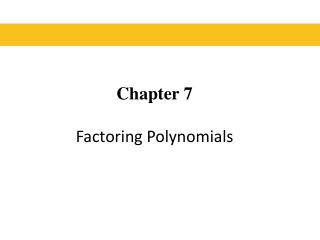# Chapter 7 Factoring Polynomials - PowerPoint PPT PresentationDownload PresentationChapter 7 Factoring Polynomials

Chapter 7 Factoring PolynomialsDownload Presentation## Chapter 7 Factoring Polynomials

- - - - - - - - - - - - - - - - - - - - - - - - - - - E N D - - - - - - - - - - - - - - - - - - - - - - - - - - -
##### Presentation Transcript

1. Chapter 7Factoring Polynomials

2. § 7.1 The Greatest Common Factor and Factoring by Grouping

3. Factoring Factoring a polynomial means finding an equivalent expression that is a product. For example, when we take the polynomial And write it as we say that we have factored the polynomial. In factoring, we write a sum as a product. Blitzer, Introductory Algebra, 5e – Slide #3 Section 7.1

4. Factoring Blitzer, Introductory Algebra, 5e – Slide #4 Section 7.1

5. Factoring Greatest Common Factor - GCF • The greatest common factor is an expression of the highest degree that divides each term of the polynomial. Blitzer, Introductory Algebra, 5e – Slide #5 Section 7.1

6. Factoring Factoring a Monomial from a Polynomial • Determine the greatest common factor of all terms in the polynomial. • Express each term as the product of the GCF and its other factor. • Use the distributive property to factor out the GCF. Blitzer, Introductory Algebra, 5e – Slide #6 Section 7.1

7. Greatest Common Factor Factor: 7x2 + 28x EXAMPLE The GCF of 7x2 and 28x is 7x. 7x2 + 28x = 7x·x +7x·4 = 7x(x + 4) Determine the GCF. Express each term as the product of the GCF and the its other factor. Factor out the GCF. Blitzer, Introductory Algebra, 5e – Slide #7 Section 7.1

8. Greatest Common Factor Factor: 5x4y - 10x2y2 + 25xy EXAMPLE The GCF of 5x4y, -10x2y2 and 25xy is 5xy. 5x4y - 10x2y2 + 25xy = 5xy·x3 - 5xy·2xy + 5xy·5 = 5xy(x3 – 2xy + 5) Determine the GCF. Express each term as the product of the GCF and the its other factor. Factor out the GCF. Blitzer, Introductory Algebra, 5e – Slide #8 Section 7.1

9. Factoring EXAMPLE Factor: SOLUTION First, we determine the greatest common factor of the three terms. Notice that the greatest integer that divides into 49, 70 and 35 (the coefficients of the terms) is 7. The variables raised to the smallest exponents are The GCF is Blitzer, Introductory Algebra, 5e – Slide #9 Section 7.1

10. Factoring CONTINUED Express each term as the product of the GCF and its other factor Factor out the GCF Blitzer, Introductory Algebra, 5e – Slide #10 Section 7.1

11. Factoring EXAMPLE Factor: SOLUTION The GCF is 5x. Because the leading coefficient, -5, is negative, we factor out a common factor with a negative coefficient. We will factor out the negative of the GCF, or -5x. Express each term as the product of the GCF and its other factor Factor out the GCF Blitzer, Introductory Algebra, 5e – Slide #11 Section 7.1

12. Factoring by Grouping EXAMPLE Factor: SOLUTION Let’s identify the common binomial factor in each part of the problem. The GCF, a binomial, is x + y. The GCF, a binomial, is x + y. Blitzer, Introductory Algebra, 5e – Slide #12 Section 7.1

13. Factoring by Grouping CONTINUED We factor out the common binomial factor as follows. This step, usually omitted, shows each term as the product of the GCF and its other factor, in that order. Factor out the GCF Blitzer, Introductory Algebra, 5e – Slide #13 Section 7.1

14. Factoring by Grouping Blitzer, Introductory Algebra, 5e – Slide #14 Section 7.1

15. Factoring by Grouping In this section, we will be usinggrouping to factor a polynomial having four terms and grouping the 4 terms into two pairs, where the pairs share a factor. • Group terms that have a common monomial factor. • Factor out the common monomial factor from each group. • Factor out the remaining common binomial factor (if one exists). Blitzer, Introductory Algebra, 5e – Slide #15 Section 7.1

16. Factoring by Grouping Factor 3x2 + 6x + 4x + 8 by grouping. EXAMPLE 3x2 + 6x + 4x + 8 = (3x2 + 6x) + (4x + 8) = 3x(x + 2) + 4(x + 2) = (x + 2)(3x + 4) Group terms that have a common monomial factor. Factor out the common monomial factor from each group. Factor out the remaining common binomial factor. Blitzer, Introductory Algebra, 5e – Slide #16 Section 7.1

17. Factoring by Grouping Factor 5x2 - xy + 15xy– 3y2 EXAMPLE 5x2 - xy + 15xy– 3y2 = (5x2 - xy) + (15xy – 3y2) = x(5x - y) + 3y(5x - y) = (5x - y)(x + 3y) Group terms that have a common monomial factor. Factor out the common monomial factor from each group. Factor out the remaining common binomial factor. Blitzer, Introductory Algebra, 5e – Slide #17 Section 7.1

18. Factoring by Grouping EXAMPLE Factor: SOLUTION There is no factor other than 1 common to all terms. However, we can group terms that have a common factor: + Common factor is : Use -2b, rather than 2b, as the common factor: -2bx – 4by = -2b(x + 2y). In this way, the common binomial factor, x + 2y, appears. Blitzer, Introductory Algebra, 5e – Slide #18 Section 7.1

19. Factoring by Grouping CONTINUED It is sometimes necessary to use a factor with a negative coefficient to obtain a common binomial factor for the two groupings. We now factor the given polynomial as follows: Group terms with common factors Factor out the common factors from the grouped terms Factor out the GCF Blitzer, Introductory Algebra, 5e – Slide #19 Section 7.1